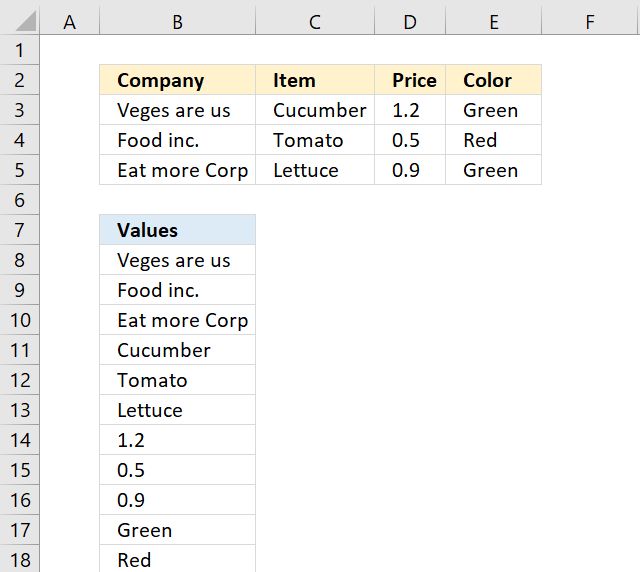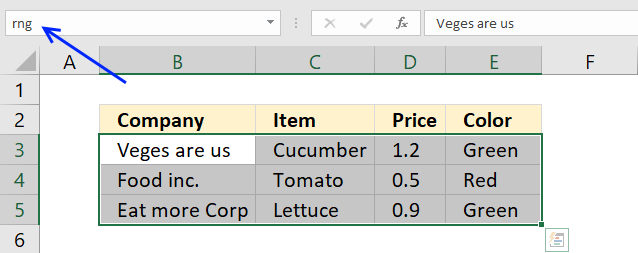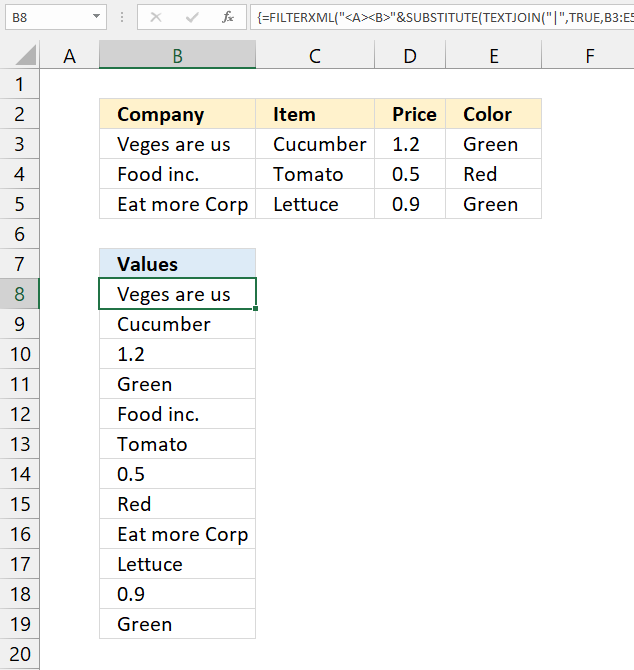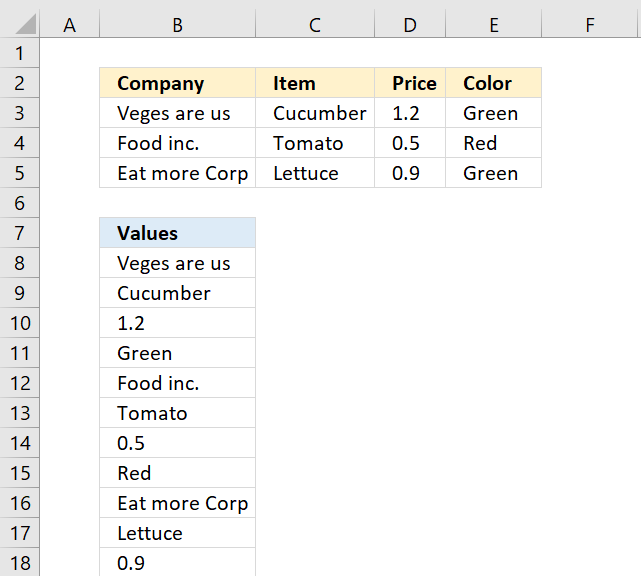Author: Oscar Cronquist Article last updated on February 01, 2023This article demonstrates formulas that rearrange values in a cell range to a single column.

## 1. Rearrange cells in a cell range to vertically distributed values (Excel formula)The formula in cell B8 uses a named range to calculate the row and column needed to extract the correct value.

=INDEX(rng, MOD(ROW(A1)-1, ROWS(rng))+1, QUOTIENT(ROW(A1)-1, ROWS(rng))+1)

rng is a named range and refers to cell range B3:E5.

Excel 365 dynamic array formula:

=TOCOL(rng, , TRUE)

The TOCOL function is a newer function available to Excel 365 subscribers, it lets you rearrange values in a 2D cell range to a single column.

TOCOL(array, [ignore], [scan_by_col])

Use the following Excel 365 function to distribute values horizontally:

=TOROW(rng, , TRUE)

The TOROW function rearranges values from a 2D cell range to a single row.

TOROW(array, [ignore], [scan_by_col])

## 2. How to quickly create a named rangeThis step is optional, you can use just as well use a cell reference.

To create a named range simply select the cell range (B3:E5) and press with left mouse button on in the name box. Type a name for that range, I named the cell range rng. Press Enter, that's it.

## 3. Explaining formula in cell B8

#### Step 1 - Count rows in cell range

The INDEX function needs a row and column number in order to get the correct value.

The ROWS function returns the number of rows in a cell range.

ROWS(rng) becomes ROWS(B3:E5) and returns 3.

#### Step 2 - Create a sequence

The ROW function returns the row number of a cell reference.

ROW(A1) returns 1.

#### Step 3 - Create a repeating number sequence

To calculate the row number I use the MOD function to build a repeating number sequence. There are three rows in B3:E5 so the sequence must be 1,2,3,1,2,3, ... and so on.

MOD(ROW(A1)-1, ROWS(rng))+1

becomes

MOD(1-1, 3)+1

becomes

MOD(0, 3) + 1 and returns 1.

#### Step 4 - Create a repeating number sequence for columns

To calculate the column number I use the QUOTIENT function to build a repeating number sequence: 1,1,1,2,2,2,3 ... and so on.

QUOTIENT(ROW(A1)-1, ROWS(rng))+1

becomes

QUOTIENT(1-1, 3)+1

becomes

QUOTIENT(0, 3)+1 and returns 1.

#### Step 5 - Get value based on row and column numbers

The INDEX function then returns the value in row 1 and column 1 from cell range 3:E5.

INDEX(rng, MOD(ROW(A1)-1, ROWS(rng))+1, QUOTIENT(ROW(A1)-1, ROWS(rng))+1)

becomes

INDEX(rng, 1, 1)

and returns "Veges are us" in cell B8.

## 4. Rearrange cells in a cell range to vertically distributed values (Excel 2019 formula)Array formula in cell B8:

=FILTERXML("<A><B>"&SUBSTITUTE(TEXTJOIN("|", TRUE, B3:E5), "|","</B><B>")&"</B></A>","//B")

Note, enter this formula as a regular formula if you are an Excel 365 user.

## 5. Explaining array formula (Excel 2019)

#### Step 1 - Join values using a delimiting character

The TEXTJOIN function joins values from a cell range or array, you need at least the Excel 2019 version.

TEXTJOIN(delimiter, ignore_empty, text1, [text2], ...)

I recommend using a delimiting character that is not in cell range B3:E5 to avoid confusion.

TEXTJOIN("|", TRUE, B3:E5)

becomes

TEXTJOIN("|",TRUE,{"Veges are us","Cucumber",1.2,"Green";"Food inc.","Tomato",0.5,"Red";"Eat more Corp","Lettuce",0.9,"Green"})

and returns

"Veges are us|Cucumber|1.2|Green|Food inc.|Tomato|0.5|Red|Eat more Corp|Lettuce|0.9|Green"

#### Step 2 - Substitute delimiting character with XML tag

The SUBSTITUTE function substitutes a specific text string in a value.

SUBSTITUTE(text, old_text, new_text, [instance_num])

SUBSTITUTE(TEXTJOIN("|", TRUE, B3:E5), "|","</B><B>")

becomes

SUBSTITUTE("Veges are us|Cucumber|1.2|Green|Food inc.|Tomato|0.5|Red|Eat more Corp|Lettuce|0.9|Green",  "|","</B><B>")

and returns

"Veges are us</B><B>Cucumber</B><B>1.2</B><B>Green</B><B>Food inc.</B><B>Tomato</B><B>0.5</B><B>Red</B><B>Eat more Corp</B><B>Lettuce</B><B>0.9</B><B>Green"

#### Step 3 - Concatenate string with XML tag

The ampersand character & lets you concatenate strings in an Excel formula.

"<A><B>"&SUBSTITUTE("Veges are us|Cucumber|1.2|Green|Food inc.|Tomato|0.5|Red|Eat more Corp|Lettuce|0.9|Green", "|","</B><B>")&"</B></A>"

becomes

"<A><B>"&"Veges are us</B><B>Cucumber</B><B>1.2</B><B>Green</B><B>Food inc.</B><B>Tomato</B><B>0.5</B><B>Red</B><B>Eat more Corp</B><B>Lettuce</B><B>0.9</B><B>Green"&"</B></A>"

and returns

"<A><B>Veges are us</B><B>Cucumber</B><B>1.2</B><B>Green</B><B>Food inc.</B><B>Tomato</B><B>0.5</B><B>Red</B><B>Eat more Corp</B><B>Lettuce</B><B>0.9</B><B>Green</B></A>"

#### Step 4 - Extract values from XML data

The FILTERXML function extracts specific values from XML content by using the given xpath.

FILTERXML(xml, xpath)

FILTERXML("<A><B>"&SUBSTITUTE(TEXTJOIN("|", TRUE, B3:E5), "|","</B><B>")&"</B></A>","//B")

becomes

FILTERXML("<A><B>Veges are us</B><B>Cucumber</B><B>1.2</B><B>Green</B><B>Food inc.</B><B>Tomato</B><B>0.5</B><B>Red</B><B>Eat more Corp</B><B>Lettuce</B><B>0.9</B><B>Green</B></A>","//B")

and returns

{"Veges are us"; "Cucumber"; 1.2; "Green"; "Food inc."; "Tomato"; 0.5; "Red"; "Eat more Corp"; "Lettuce"; 0.9; "Green"}.

## 6. Returning values row by rowThe following formula gets the values row by row:

=INDEX(rng, QUOTIENT(ROW(A1)-1, COLUMNS(rng))+1, MOD(ROW(A1)-1, COLUMNS(rng))+1)

Excel 365 dynamic array formula:

=TOCOL(rng, , FALSE)

The TOCOL function is a newer function available to Excel 365 subscribers, it lets you rearrange values in a 2D cell range to a single column.

TOCOL(array, [ignore], [scan_by_col])